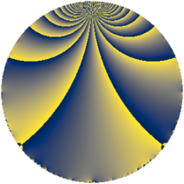# Properties

 Label 125.2.bLevel $125$ Weight $2$ Character orbit 125.b Rep. character $\chi_{125}(124,\cdot)$ Character field $\Q$ Dimension $8$ Newform subspaces $2$ Sturm bound $25$ Trace bound $4$

# Related objects

## Defining parameters

 Level: $$N$$ $$=$$ $$125 = 5^{3}$$ Weight: $$k$$ $$=$$ $$2$$ Character orbit: $$[\chi]$$ $$=$$ 125.b (of order $$2$$ and degree $$1$$) Character conductor: $$\operatorname{cond}(\chi)$$ $$=$$ $$5$$ Character field: $$\Q$$ Newform subspaces: $$2$$ Sturm bound: $$25$$ Trace bound: $$4$$ Distinguishing $$T_p$$: $$2$$

## Dimensions

The following table gives the dimensions of various subspaces of $$M_{2}(125, [\chi])$$.

Total New Old
Modular forms 18 8 10
Cusp forms 8 8 0
Eisenstein series 10 0 10

## Trace form

 $$8 q - 6 q^{4} - 4 q^{6} - 4 q^{9} + O(q^{10})$$ $$8 q - 6 q^{4} - 4 q^{6} - 4 q^{9} - 4 q^{11} + 8 q^{14} - 2 q^{16} - 10 q^{19} + 6 q^{21} + 20 q^{24} - 14 q^{26} + 10 q^{29} + 6 q^{31} + 18 q^{34} - 22 q^{36} + 2 q^{39} - 14 q^{41} - 22 q^{44} + 26 q^{46} - 6 q^{49} + 26 q^{51} - 10 q^{54} + 10 q^{56} - 30 q^{59} - 4 q^{61} - 16 q^{64} + 2 q^{66} + 32 q^{69} - 24 q^{71} - 12 q^{74} + 20 q^{76} - 40 q^{79} - 32 q^{81} + 8 q^{84} + 56 q^{86} + 30 q^{89} + 46 q^{91} - 2 q^{94} - 34 q^{96} + 2 q^{99} + O(q^{100})$$

## Decomposition of $$S_{2}^{\mathrm{new}}(125, [\chi])$$ into newform subspaces

Label Dim $A$ Field CM Traces $q$-expansion
$a_{2}$ $a_{3}$ $a_{5}$ $a_{7}$
125.2.b.a $4$ $0.998$ 4.0.4400.1 None $$0$$ $$0$$ $$0$$ $$0$$ $$q-\beta _{3}q^{2}-\beta _{1}q^{3}+(-1-2\beta _{2})q^{4}+\cdots$$
125.2.b.b $4$ $0.998$ $$\Q(i, \sqrt{5})$$ None $$0$$ $$0$$ $$0$$ $$0$$ $$q+\beta _{1}q^{2}+(\beta _{1}+2\beta _{3})q^{3}+(1+\beta _{2})q^{4}+\cdots$$